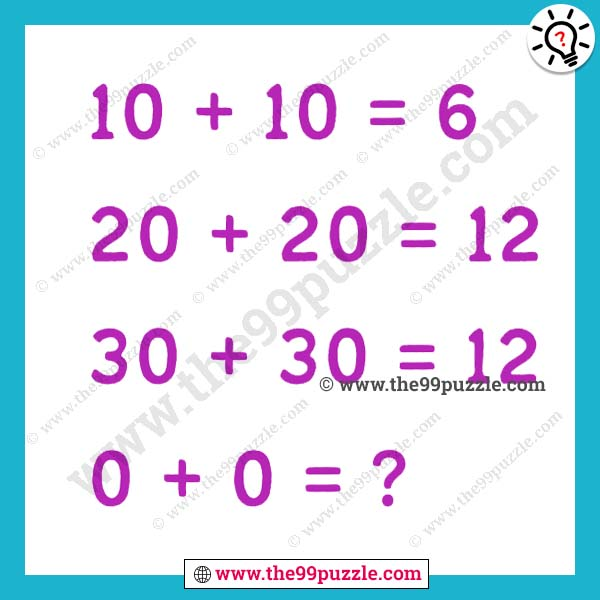# Fun tricky math puzzle for adults and kids – Puzz266

This type of viral math puzzle is very challenging and confusing. Logical thinking math equations can only solve the genius people. If you understand the logic you can easily find out the value of the logical thinking viral math equations. This fun tricky math puzzle makes you smarter if you practice every day. In this mathematics, you have to find out the math tricks and replace the question mark. Genius students solve the puzzles very quickly.

10+10=6

20+20=12

30+30=12

0+0=?###### Explanation:

The total number of letters in two numbers in each equation.

10+10=TEN+TEN = 6

20+20=TWENTY+TWENTY = 12

30+30=THIRTY+THIRTY = 12

0+0=ZERO+ZERO = 8